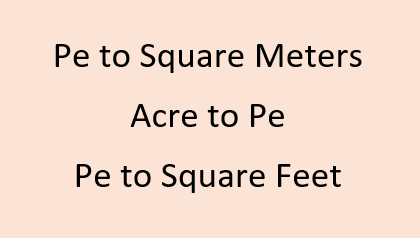# Pe to Square Meters | Acre to Pe | Pe to Square Feet## Pe to Square Meters | Acre to Pe | Pe to Square FeetPe is a traditional unit of land area measurement commonly in use in Taiwan. It is known as Phiàng in Hakka and Píng in Mandarin. It symbol is . It is the same as Japanese Tsubo. It is comparatively very small in value considering the other traditional units of area measurement in Taiwan. Being a traditional unit people want to know the value of Pe in standard units and hence they ask questions like 1 Pe is equal to how many square feet? or 1 Pe is equal to how many square meters? or 1 hectare consists of how many Pe ? or how many Pe make an acre ?
Now 1 Pe = 400/121 m² to be exact.
1 Pe = 3.305 square meters = 35.58 square feet (approx)

Convert Pe to other units of Land area measurement :
 Value Convert from Le Kah Hun Bo Pe Sq. Centimeters Sq. Feet Sq. Yards Hectares Acres Sq. Meters Sq. Kilometers Sq. Miles Cent Convert to Le Kah Hun Bo Pe Sq. Centimeters Sq. Feet Sq. Yards Hectares Acres Sq. Meters Sq. Kilometers Sq. Miles Cent

Area Conversion of Pe to other Units and vice versa:
1 Pe = 0.000816877294106332 Acre
1 Pe = 0.000330578512396694 Hectare
1 Pe = 35.5831749312718 Sq. Feet
1 Pe = 3.30578512396694 Sq. Meter
1 Pe = 3.30578512396694E-06 Sq. Kilometer
1 Pe = 3.95368610347465 Sq. Yard
1 Pe = 0.0816877294106332 Decimal
1 Pe = 6.81663258350375E-05 Le
1 Pe = 0.000340831629175187 Kah
1 Pe = 0.00340831629175188 Hun
1 Pe = 0.0333333333333333 Bo
1 Le = 14670 Pe
1 Kah = 2934 Pe
1 Hun = 293.4 Pe
1 Bo = 30 Pe
1 Acre = 1224.174067776 Pe
1 Hectare = 3025 Pe
1 Sq. Feet = 0.0281031696 Pe
1 Sq. Meter = 0.3025 Pe
1 Sq. Kilometer = 302500 Pe
1 Sq. Yard = 0.2529285264 Pe
1 Decimal = 12.24174067776 Pe

Q. 1 Acre consists of how many Pe ?
A. 1 Acre consists of approx 1224.17 Pe

Q. Convert Pe to Square Yards
A. 1 Pe is equal to 3.95368610347465 Sq. Yards

Q. Pe to other traditional units of land measurement
A. 1 Bo = 30 Pe and 1 Hun = 293.4 Pe and 1 Kah = 2934 Pe and 1 Le = 14670 Pe

Q. 1 Pe is how many sq feet?
A. 1 Pe = 35.5831749312718 Sq. Feet

Q. How many Pe make an Hectare (ha)?
A. 1 Hectare = 3025 Pe